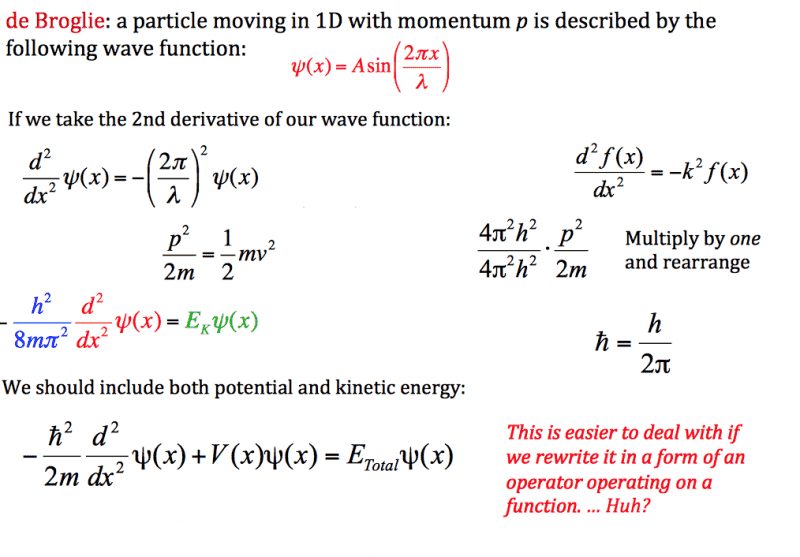# Question about the Hamiltonian Operator

• plazprestige

#### plazprestige

In my physical chemistry course, we are learning about the Schrödinger Equation and were introduced to the Hamiltonian Operator recently. We started out with the simple scenario of a particle in 1D space. Our professor's slide showed the following "derivation" to arrive at the expression for the kinetic energy component of the Hamiltonian (see attachment).I do not understand how the professor arrived at the eigenfunction-eigenvalue equation for the kinetic energy involving Planck's constant. I understand that kinetic energy is equal to momentum squared divided by twice the mass, of course, but nothing beyond that in the slide. If someone could explain this, I would appreciate it. Please note that I am not a physics major, so any mathematics more advanced than calculus may be beyond me (I do understand operators and eigenfunctions, however).

Its basically hand-wavey rubbish given so you basically don't tell students its pulled out of a hat.

The reason for Schrodingers equation believe it or not is the principle of relativity of classical mechanics ie time is invariant. But proving so involves advanced math. You will find the derivation in Ballentine - Quantum Mechanics - A Modern Development - Chapter 3. At your level you probably won't understand it, but I do suggest you get a copy from your university library and have a look at it to get a bit of its gist.

As to how Schroedinger did it, which what your professor wrote is reminiscent of, check out:
http://arxiv.org/abs/1204.0653

But again its math is likely a bit beyond your level.

At your level I would have a look at the first two chapters of Griffiths - Introduction To Quantum mechanics.

I found the following that may help:

Thanks
Bill

Last edited:
The slide is very convoluted. I believe what he is doing is the following:
$$\frac{d^2 \psi}{dx^2}=-\left(\frac{2\pi}{\lambda}\right)^2 \psi$$
Multiple both sides by $\frac{h^2}{8\pi^2 m}$
$$\frac{h^2}{8\pi^2 m}\frac{d^2 \psi}{dx^2}=-\frac{h^2}{8\pi^2 m}\left(\frac{2\pi}{\lambda}\right)^2 \psi$$
Remember that $p=\frac{h}{\lambda}$ so that the equation now can be written
$$-\frac{h^2}{8\pi^2 m}\frac{d^2 \psi}{dx^2}=\frac{4 \pi^2 }{8\pi^2 m}\left(\frac{h}{\lambda}\right)^2 \psi \\ -\frac{h^2}{8\pi^2 m}\frac{d^2 \psi}{dx^2}=\frac{1}{2 m}p^2 \psi$$
Now you see the right side is the definition of kinetic energy.

I concur with Bill's first sentence.

[EDIT: fixed what Bill pointed out in the next post]

Last edited:
•bhobba
Well spotted - but there is a minor algebraical error in the third equation - an extra h^2.

I generally don't worry about such things because its a good exercise spotting them - I make errors like that all the time - and I have to say its gotten worse as I get older.

Thanks
Bill

Last edited:
•Haborix
Ah, thanks! I made that error twice, but I only caught one before posting.

All errors are unspecified exercises for the reader.

•vanhees71 and bhobba
Thank you both for your in-depth explanations. I figured that the professor was hand-waving, but it is nice to have at least some rudimentary idea of where the Hamiltonian (or the kinetic energy component) comes from. To Bill, I am familiar with the basics of Einstein's relativity, so I would be interested in hearing more about how Schrödinger's equation can be derived from that concept. If the math is way beyond calculus, then I suppose I won't worry about it.

To Bill, I am familiar with the basics of Einstein's relativity, so I would be interested in hearing more about how Schrödinger's equation can be derived from that concept. If the math is way beyond calculus, then I suppose I won't worry about it.

The math isn't beyond calculus - but is pretty heavy going. I have an degree in math and for me it was slow going.

To see if you want to tackle it see the proof of a key theorem it uses, but the proof is left out:
http://arxiv.org/pdf/0808.0779v2.pdf

If you can understand that then Ballentine should be doable - in which case see chapter 3 - Ballentine.

In math when someone says the math is a bit advanced and best left until later often it refers to this mathematical maturity thing - it quite possibly means while you may know the necessary math to understand it, ploughing through it is hard going and is best left until you have more experience with proofs of that complexity.

Thanks
Bill

Last edited: